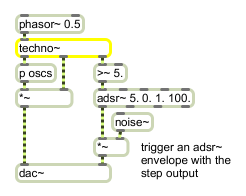# techno~

Signal-driven step sequencer

## Description

techno~ is a signal-based step-sequencer that facilitates sample-accurate timing of events.

None.

## Messages

 amplitude sequencer-step [int]amplitude-value [float] The word amplitude, followed by a number that specifies the sequencer step and a float that specifies an amplitude value, sets the amplitude (as an absolute factor) of a step's output note. The amplitude is specified as an absolute factor of that step's note -- an amplitude of 1.0 will result in the amplitude output signal having a value of 1.0 at the very beginning of the step. attack sequencer-step [int]attack-curve-exponent [float] The word attack, followed by a number that specifies the sequencer step and a float that specifies the exponent of a curve, sets the curve used to calculate the amplitude trajectory from 0.0 at the beginning of the previous step to the amplitude value at the beginning of the current step. The values used to specify the exponents of the curve are the same as those used for the curve message. curve sequencer-step [int]curve-exponent [float] The word curve, followed by a number that specifies the sequencer step and a float that specifies the exponent of a curve, sets the curve used to calculate the trajectory of pitch from the previous step. A value of 1.0 represents a linear slide from the previous step; a value of 0.5 represents a square root function; a value of 2.0 represents a second-order parabolic slide; etc. The curve message lets you set and experiment with different varieties of portamento. decay sequencer-step [int]decay-trajectory-exponent [float] The word decay, followed by a number that specifies the sequencer step and a float that specifies the exponent of a curve, sets the curve used to calculate the decay trajectory from the amplitude value at the beginning of this previous step to 0.0 at the beginning of the next step. The values used to specify the exponents of the curve are the same as those used for the curve message. length number-of-sequenced-notes (steps) [int] The word length, followed by a number, sets the number of notes in the sequence. The default is 1. pitch sequencer-step [int]fequency (Hz) [float] The word pitch, followed by a number that specifies the sequencer step and a float that specifies a pitch as a Hertz frequency, sets the pitch of that step's note. pos sequencer-step [int]start-position (step-size-coefficient) [float] The word pos, followed by an integer that specifies the sequencer step and a float that specifies a start position, positions the step to the specified position. A step may not be placed before the previous step or after the next step. For instance, a uniformly-spaced four step sequence will have its steps in positions 0.0, 0.25, 0.5 and 0.75, so a pos message for the third step (index 2) can only specify positions between 0.25 and 0.75. signal A signal is used as an input to the techno~ object to specify the step position in a sequence. The signal is in the range 0 - 1.0 and indicates a phase value, expressed as a fraction of the number of total steps in the sequence (set using the length message). A phasor~ object is customarily used as input to the techno~ object. All input signals are clipped to the range 0 - 1.0. repeatpos step-width [list] The word repeatpos, followed by one or more floats, allows repeating settings of non-uniform sequencer step sizes. The number of floats following the lengths message represents one less than the size of the repeating segment of steps - this segment size can be any even divisor of the total number of steps in the sequence. So for instance with an eight-step sequence the length of the segment can be 2, 4, or 8 steps. The floating point arguments, which must be strictly increasing and in the range between 0 and 1, set the relative width of each step. For instance, one can set uniform divisions for a sequence with an even number of steps with any of the following messages: repeatpos 0.5 repeatpos 0.25 0.5 0.75 repeatpos 0.125 0.25 0.375 0.5 0.625 0.75 0.875 The message repeatpos 0.66 affects a repeating segment two steps long, giving the first step 66% of the time and the second step 34%. (This is like classic "swing" on a drum machine.)

## Output

signal: Out left inlet: Pitch signal output for oscillator(s).

Out middle inlet: An amplitude envelope. You can multiply this signal output with the output of your oscillators.

Out right inlet: The current position in the step sequence. Each step represents a distance of 1.0 and the total output range is from 0 to the value set by the size message.

## Examplestechno~ use as a synth sequencer or to trigger individual samples like a drum machine